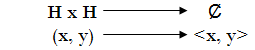### Hilbert Spaces

A Vectorial Space H, is a Normed Space if it can define the operation we call Norm , that satisfies

1) ||x|| ≥ 0, ||x|| = 0 <=> x=0, ∀x∈ H

2) ||kx|| = |k| ||x|| = 0, ∀x∈ H, ∀k∈C

3) ||x +y|| ≤ ||x|| + ||y||, ∀x, y∈ H

This last inequality is called the triangle inequality.
A vectorial space H, with the operationis said is Pre-Hilbert if it satisfyes

1) <x, x> ≥ 0, <x, x> = 0 <=> x=0, ∀x∈ H

1) <kx, y> = k<x, y> ∀x, y∈ H , ∀k∈C

3) <x, y> = <y, x>

4) <x1 + x2, y> = <x1, y> + <x2, y>

A Pre-Hilbert space is said to be a Hilbert space if is Banach space (normed and complete) with the norm generating.

All Hilbert space is a normed space if we consider the norm generated by

||x|| = <x, x>1/2

So, we have the next reciprocal:
Every Banach space is Hilbert if it satisfies the parallelogram identity

||x + y||2 + ||x - y||2 = 2(||x||2 + ||y||2)

Because we can consider the inner product given by the polarization identity

4<x, y> = ||x + y||2 + ||x - y||2 -i ( ||x + iy||2 + ||x - iy||2 )

An important aspect of a Hilbert space is that it meets theCauchy-Schwarz inequality

<x, y> ≤ ||x|| ||y||